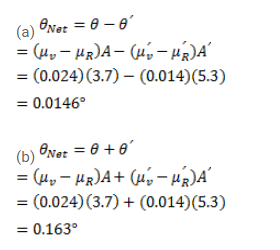# The refractive index of a material M1 changes byQuestion:

The refractive index of a material M1 changes by $0.014$ and that of another material M2 changes by $0.024$ as the colour of the light is changed from red to violet. Two thin prisms one made of $M 1\left(A=5.3^{\circ}\right)$ and other made of $\mathrm{M} 2\left(\mathrm{~A}=3.7^{\circ}\right)$ are combined with their reflecting angles oppositely directed.

(a) Find the angular dispersion produced by the combination.

(b) The prisms are now combined with their refracting angles similarly directed. Find the angular dispersion produced by the combination.

Solution: